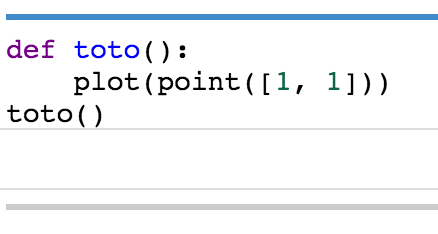# Plot in a function

Hello,

Is it normal that i can't plot in a function ?

Without a function:

plot(point([1, 1]))With a function:

def toto():
plot(point([1, 1]))
toto()edit retag close merge delete

It is better to write the code instead of providing picture. If I want to try the code it is simpler for me to do a copy paste... that is not possible with pictures.

Sort by » oldest newest most voted

First of all in your code you can replace plot(point([1,1])) by point([1,1]).

And the answer is yes. All plot functions return a graphic objects that can be further manipulated. In your function you are creating a graphic but not using it at all. The following code shows what is a graphic

sage: G = point([1,1])
sage: type(G)
<class 'sage.plot.graphics.Graphics'>
sage: G    # will show the graphics


If you want that your function shows the graphic you can do

def my_graphic():
G = point([1,1])
G.show()


If you want that your function returns a graphic you can do

def my_graphic():
G = point([1,1])
return G

more

Thanks ! If I call several times the function, how can I concatenate the graphics to show them in one graph ?

sage: point([1,1]) + point([3,2])